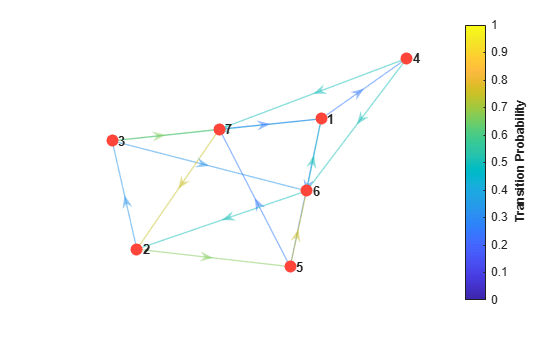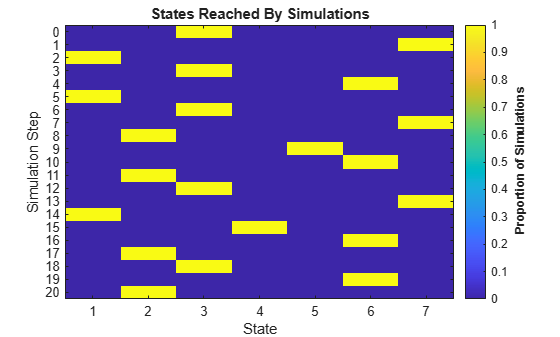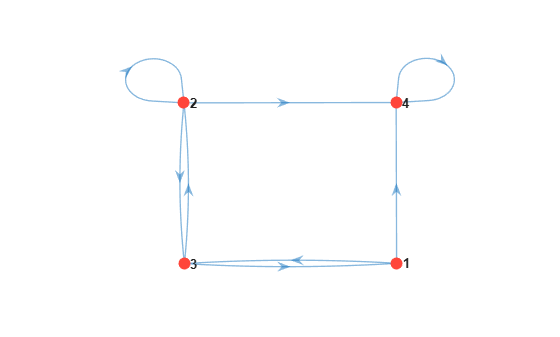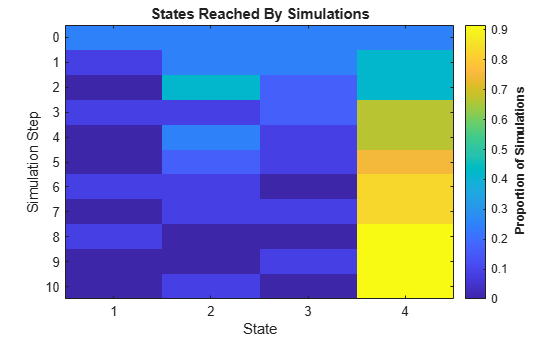Documentation

simulate

Simulate Markov chain state walks

Description

example

X = simulate(mc,numSteps) returns data X on random walks of length numSteps through sequences of states in the discrete-time Markov chain mc.

example

X = simulate(mc,numSteps,'X0',x0) optionally specifies the initial state of simulations x0.

Examples

collapse all

Consider this theoretical, right-stochastic transition matrix of a stochastic process.

$P=\left[\begin{array}{ccccccc}0& 0& 1/2& 1/4& 1/4& 0& 0\\ 0& 0& 1/3& 0& 2/3& 0& 0\\ 0& 0& 0& 0& 0& 1/3& 2/3\\ 0& 0& 0& 0& 0& 1/2& 1/2\\ 0& 0& 0& 0& 0& 3/4& 1/4\\ 1/2& 1/2& 0& 0& 0& 0& 0\\ 1/4& 3/4& 0& 0& 0& 0& 0\end{array}\right].$

Create the Markov chain that is characterized by the transition matrix P.

P = [ 0   0  1/2 1/4 1/4  0   0 ;
0   0  1/3  0  2/3  0   0 ;
0   0   0   0   0  1/3 2/3;
0   0   0   0   0  1/2 1/2;
0   0   0   0   0  3/4 1/4;
1/2 1/2  0   0   0   0   0 ;
1/4 3/4  0   0   0   0   0 ];
mc = dtmc(P);

Plot a directed graph of the Markov chain. Indicate the probability of transition by using edge colors.

figure;
graphplot(mc,'ColorEdges',true);Simulate a 20-step random walk that starts from a random state.

rng(1); % For reproducibility
numSteps = 20;
X = simulate(mc,numSteps)
X = 21×1

3
7
1
3
6
1
3
7
2
5
⋮

X is a 21-by-1 matrix. Rows correspond to steps in the random walk. Because X(1) is 3, the random walk begins at state 3.

Visualize the random walk.

figure;
simplot(mc,X);Create a four-state Markov chain from a randomly generated transition matrix containing eight infeasible transitions.

rng('default'); % For reproducibility
mc = mcmix(4,'Zeros',8);

mc is a dtmc object.

Plot a digraph of the Markov chain.

figure;
graphplot(mc);State 4 is an absorbing state.

Run three 10-step simulations for each state.

x0 = 3*ones(1,mc.NumStates);
numSteps = 10;
X = simulate(mc,numSteps,'X0',x0);

X is an 11-by-12 matrix. Rows corresponds to steps in the random walk. Columns 1–3 are the simulations that start at state 1; column 4–6 are the simulations that start at state 2; columns 7–9 are the simulations that start at state 3; and columns 10–12 are the simulations that start at state 4.

For each time, plot the proportions states that are visited over all simulations.

figure;
simplot(mc,X)Input Arguments

collapse all

Discrete-time Markov chain with NumStates states and transition matrix P, specified as a dtmc object. P must be fully specified (no NaN entries).

Number of discrete time steps in each simulation, specified as a positive integer.

Data Types: double

Name-Value Pair Arguments

Specify optional comma-separated pairs of Name,Value arguments. Name is the argument name and Value is the corresponding value. Name must appear inside quotes. You can specify several name and value pair arguments in any order as Name1,Value1,...,NameN,ValueN.

Example: 'X0',[1 0 2] specifies simulating three times, the first simulation starts in state 1 and the final two start in state 3.

Initial states of simulations, specified as the comma-separated pair consisting of 'X0' and a vector of nonnegative integers of NumStates length. X0 provides counts for the number of simulations to begin in each state. The total number of simulations (numSims) is sum(X0).

The default is a single simulation beginning from a random initial state.

Example: 'X0',[10 10 0 5]

Data Types: double

Output Arguments

collapse all

Indices of states visited during the simulations, returned as a (1 + numSteps)-by-numSims numeric matrix of positive integers. The first row contains the initial states. Columns, in order, are all simulations beginning in the first state, then all simulations beginning in the second state, and so on.

Tips

• To start n simulations from state k, use:

X0 = zeros(1,NumStates);
X0(k) = n;

• To visualize the data created by simulate, use simplot.